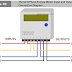Energy Meter is a very useful and important measuring instrument. The energy meter is a device by which we can measure electrical energy. The energy meter is Integrating measuring Instrument. There are two types of energy meters according to phases - 1. Single Phase Energy Meter and 2. Three Phase Energy Meter. Today we are going to see the Three(3) phase energy meter connection diagram. Also, we will know the three-phase energy meter wiring procedure with power supply and loads.

## 3-phase energy meter terminals

Before going to make the connection we must know the input and output terminals of the 3-phase energy meter. It has a total of 8 terminals where 4 terminals are for input and the other 4 terminals are for output. Count terminals from your left side to write-side.
• Terminal '1'- Input of 'R' phase
• Terminal '2'- Output of 'R' phase
• Terminal '3'- Input of 'Y' phase
• Terminal '4'- Output of 'Y' phase
• Terminal '5'- Input of 'B' phase
• Terminal '6'- Output of 'B' phase
• Terminal '7'- Input of Neutral
• Terminal '8'- Output of Neutral

## Three(3) Phase Energy Meter Connection Diagram

Here, you can three-phase energy meter input and output connection diagram.

As we know that there are four terminals for each input and output which includes Three Phases(R, Y, B) and a Neutral. Sometimes we need to connect the neutral wire and sometimes we don't need a neutral connection. So, here we will see both types of connections where neutral wire need or not.

### 3 Phase Energy Meter Connection Diagram with Load(with Neutral Wire)

Here, you can see the connection diagram of a three-phase energy meter with a load that uses the neutral wire.

Wiring Procedure
1. The 'R' Phase or Line 1 from the power source is to be connected to the Terminal 1 of the energy meter.
2. The 'Y' Phase or Line 2 from the power source is to be connected to the Terminal 3 of the energy meter.
3. The 'B' Phase or Line 3 from the power source is to be connected to the Terminal 5 of the energy meter.
4. The neutral terminal from the power source is to be connected to the Terminal 7 of the energy meter.
5. The 'R' Phase or Line 1 of the load is to be connected to the Terminal 2 of the energy meter.
6. The 'Y' Phase or Line 2 of the load is to be connected to the Terminal 4 of the energy meter.
7. The 'B' Phase or Line 3 of the load is to be connected to the Terminal 6 of the energy meter.
8. The neutral terminal of the load is to be connected to the Terminal 8 of the energy meter.

### 3 Phase Energy Meter connection diagram with a 3 phase motor(without Neutral)

Many people become confused when they are going to connect the three-phase energy with the load which does not need Neutral. There are some 3-phase energy meters that do not need any neutral connection when used with a three-phase three-wire load but some 3-phase energy meters are there that need the neutral connection even the load does not need the neutral connection. So read the user manual of your 3-phase energy meter provided by the manufacturer company when you going to connect.

As we know most of the three-phase motors do not need neutral for their operation. So, here you can see we just connected three phases only. Practically, there is no application where a three-phase motor is to be directly connected to an energy meter. Here, just an example that if neutral is not connected with the load then also energy meter can measure. Here, you can see we have connected the neutral wire at the input side only as our energy meter needs.

Wiring Procedure
1. Connect the 'R' Phase or Line 1 from the power source to the Terminal 1 of the energy meter.
2. Connect the 'Y' Phase or Line 2 from the power source to the Terminal 3 of the energy meter.
3. Connect the 'B' Phase or Line 1 from the power source to the Terminal 5 of the energy meter.
4. The neutral terminal from the power source is to be connected to the Terminal 7 of the energy meter. If your energy meter doesn't need the neutral connection for a three-phase three-wire load then keep it disconnected.
5. The 'R' Phase or Line 1 of the load is to be connected to the Terminal 2 of the energy meter.
6. The 'Y' Phase or Line 2 of the load is to be connected to the Terminal 4 of the energy meter.
7. The 'B' Phase or Line 3 of the load is to be connected to the Terminal 6 of the energy meter.
8. Keep disconnected terminal 8 of the energy meter as we don't need the neutral connection.

Thank you for visiting the website. keep visiting for more updates.

Three(3) Phase Energy Meter Connection Diagram and WiringReviewed by Author on October 30, 2018 Rating: 5